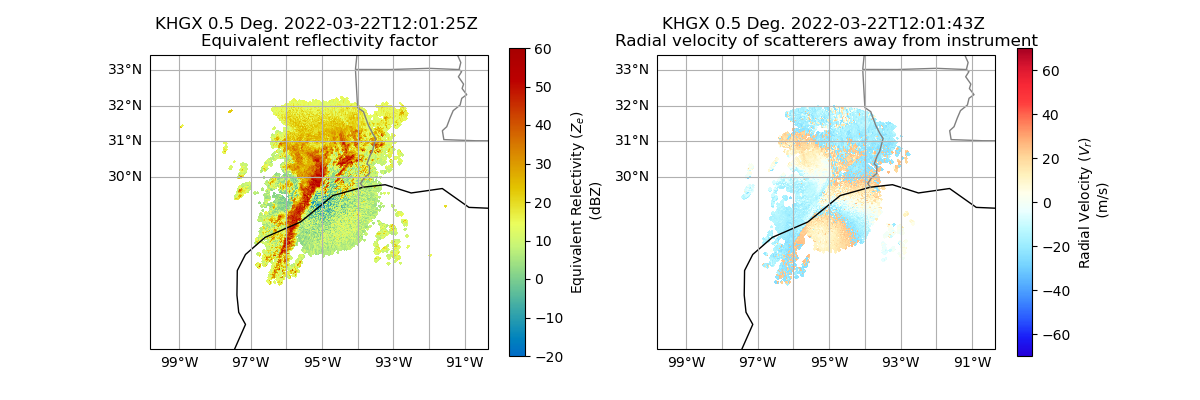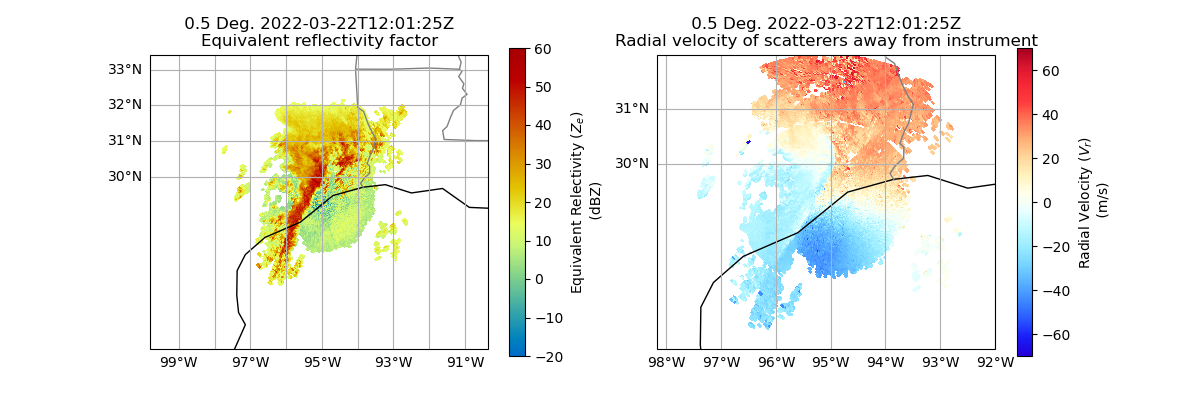Within this example, we show how you can remotely access Next Generation Weather Radar (NEXRAD) Data from Amazon Web Services and plot quick looks of the datasets.

```print(__doc__)

# Author: Max Grover (mgrover@anl.gov)

import cartopy.crs as ccrs
import matplotlib.pyplot as plt

import pyart
```

Let’s start first with NEXRAD Level 2 data, which is ground-based radar data collected by the National Oceanic and Atmospheric Administration (NOAA), as a part of the National Weather Service ### Configure our Filepath for NEXRAD Level 2 Data We will access data from the noaa-nexrad-level2 bucket, with the data organized as:

`s3://noaa-nexrad-level2/year/month/date/radarsite/{radarsite}{year}{month}{date}_{hour}{minute}{second}_V06`

Where in our case, we are using a sample data file from Houston, Texas (KHGX) on March 22, 2022, at 1201:25 UTC. This means our path would look like:

```aws_nexrad_level2_file = (
)
```

We can use the pyart.io.read_nexrad_archive module to access our data, passing in the filepath.

```radar = pyart.io.read_nexrad_archive(aws_nexrad_level2_file)
```

Let’s take a look at a summary of what fields are available.

```list(radar.fields)
```
```['velocity', 'differential_phase', 'cross_correlation_ratio', 'spectrum_width', 'clutter_filter_power_removed', 'differential_reflectivity', 'reflectivity']
```

Let’s plot the reflectivity/velocity fields as a first step to investigating our dataset.

Note: the reflectivity and velocity fields are in different sweeps, so we will need to specify which sweep to plot in each plot.

```fig = plt.figure(figsize=(12, 4))

ax = plt.subplot(121, projection=ccrs.PlateCarree())

display.plot_ppi_map(
"reflectivity",
sweep=0,
ax=ax,
colorbar_label="Equivalent Relectivity (\$Z_{e}\$) \n (dBZ)",
vmin=-20,
vmax=60,
)

ax = plt.subplot(122, projection=ccrs.PlateCarree())

display.plot_ppi_map(
"velocity",
sweep=1,
ax=ax,
vmin=-70,
vmax=70,
)
```Within this plot, we see that the velocity data still has regions that are folded, indicating the dataset has not yet been dealiased.

We can also access NEXRAD Level 3 data using Py-ART!

Each Level 3 data field is stored in separate file - in this example, we will look at the reflectivity and velocity field at the lowest levels. These correspond to the following variable names:

• `N0U` - Velocity at the lowest level

• `NOQ` - Reflectivity at the lowest level

These datasets are also in a different bucket (unidata-nexrad-level3), and the files are in a flat directory structure using the following naming convention:

`s3://unidata-nexrad-level3/{radarsite}_{field}_{year}_{month}_{date}_{hour}_{minute}_{second}`

For example, we can look at data from that same time as the NEXRAD Level 2 data used previously (March 22, 2022 at 1201 UTC)

```aws_nexrad_level3_velocity_file = (
)
)
```

```radar_level3_velocity = pyart.io.read_nexrad_level3(aws_nexrad_level3_velocity_file)
)
```

Let’s confirm that each radar object has a single field:

```print(
)
```
```velocity radar object:  ['velocity'] reflectivity radar object:  ['reflectivity']
```

Plot a Quick Look of our NEXRAD Level 3 Data

Let’s plot the reflectivity/velocity fields as a first step to investigating our dataset.

Note: the reflectivity and velocity fields are in different radars, so we need to setup different displays.

```fig = plt.figure(figsize=(12, 4))

ax = plt.subplot(121, projection=ccrs.PlateCarree())

reflectivity_display.plot_ppi_map(
"reflectivity",
ax=ax,
colorbar_label="Equivalent Relectivity (\$Z_{e}\$) \n (dBZ)",
vmin=-20,
vmax=60,
)

ax = plt.subplot(122, projection=ccrs.PlateCarree())

velocity_display.plot_ppi_map(
"velocity",
ax=ax,